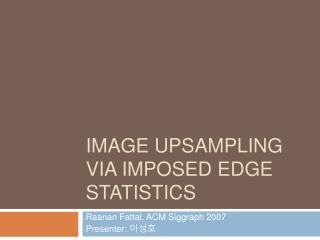DownloadDownload PresentationImage upsampling via Imposed Edge Statistics

# Image upsampling via Imposed Edge Statistics

Télécharger la présentation## Image upsampling via Imposed Edge Statistics

- - - - - - - - - - - - - - - - - - - - - - - - - - - E N D - - - - - - - - - - - - - - - - - - - - - - - - - - -
##### Presentation Transcript

1. Image upsampling via Imposed Edge Statistics RaananFattal. ACM Siggraph 2007 Presenter: 이성호

2. PreviousworkClassical approach • Nearest-Neighbor, Bilinear, Bicubic, Hann, Hamming, and Lanczos interpolation kernels. • assumption that • the image data is either spatially smooth or band-limited

3. More sophisticated methods • [Su and Willis 2004] • Reduce the number of variables that are averaged • forms a noticeable block-like effect Bicubic Su and Willis 2004

4. [Li and Orchard 2001] • Arbitrary edge orientation is implicitly matched • By estimating local intensity covariance • from the low-resolution image • Generating smooth curves and of reducing jaggies • Not sharp edges

5. [Hertzmann et al. 2001] • Image Analogies

6. [Freeman et al. 2002] • adding high-frequency patches • from a non-parametric set of examples • relating low and high resolutions • Sharpens edges and yields images with a detailed appearance • tends to introduce some irregularitiesinto the constructed image

7. [Osher et al. 2003] • invert a blurring process • measures the L1 norm of the output image

8. Assumptions on image upsampling • different upsampling techniques correspond to different assumptions: • images are smooth enough to be adequately approximated by polynomials • yields analytic polynomial-interpolation formulas • images are limited in band • yields a different family of low-pass filters • these assumptions are highly inaccurate • suffer from excessive blurriness and the other visual artifacts

9. Edge-Frame Continuity Moduli • predict the spatial intensity differences • at the high-resolution based on the low-resolution input image

10. Approach • Statistics of intensity differences • intensity conservation constraint • we discuss only gray scale images • later extend to handle color images

11. Derivatives

12. Image statistics

13. edge-frame continuity modulus (EFCM)

14. Upsampling using the EFCM

15. Gauss-Markov Random Field model

16. Color images • First we upsample the luminance channel • of the YUV color space • compute the absolute value of its luminance difference d1 d2 d3 d4

17. Results High-res original Downsampled

18. Bilinear Ours

19. Simple Edge Sensitive New Edge-Directed

20. magnified by a factor of 4

21. magnified by a factor of 8

22. magnified by a factor of 16

23. objective error measurements between an upsampled image and the original ground-truth image (i.e., before downsampling). Structural Similarity Image Quality (SSIQ) described in [Wang et al. 2004]

24. Implementations • implemented in C++ • Mobile Pentium-M, running at 2.1MHz • Upsample an image of 1282 pixels • to twice its resolution (2562). • 2 seconds • To a resolution of 10242 pixels • 22 seconds.

25. Conclusions • Drawbacks: • Emphasize lack of texture and absence of fine-details • The jaggies artifact • Acutely twisted edges • involves more computations • than some of the existing techniques • generic behavior of edges does not accurately describe every particular case. • Further improve • Using higher-order edge properties • Such as curvature

26. Appendix Numerical analysis on EFCM upsampling

27. Lagrange multipliers

28. Apply to the formula in this paper • Solve this linear system • with Conjugate Gradient-based Null Space method